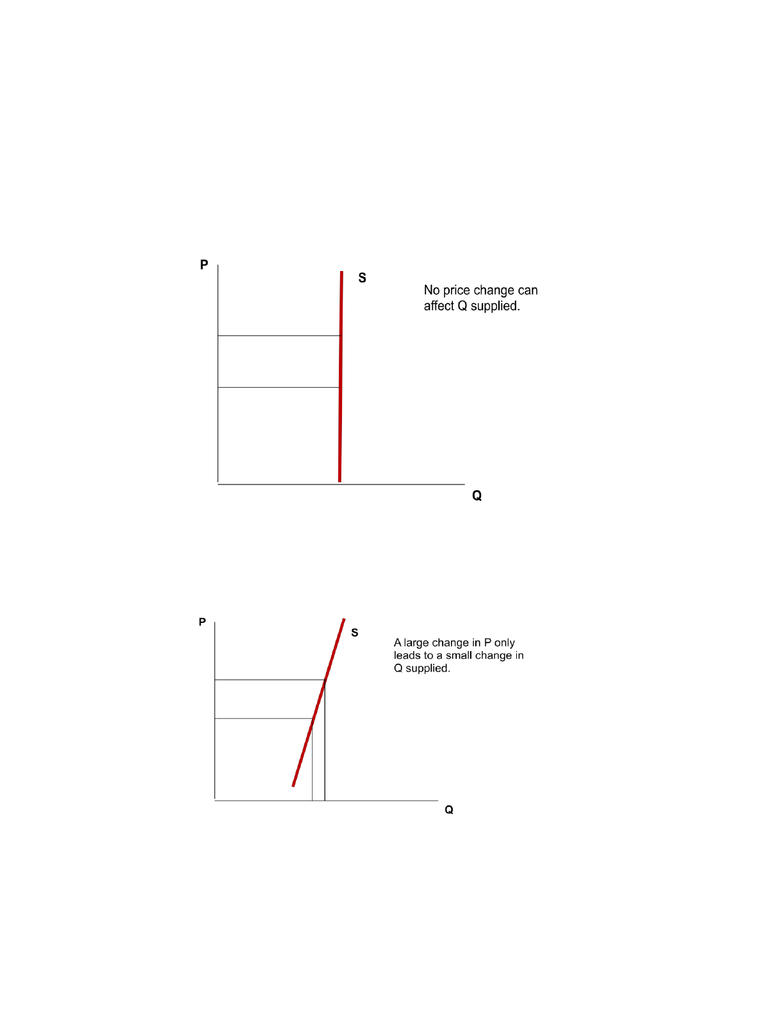Textbook Notes (280,000)
CA (160,000)
McMaster (10,000)
ECON (800)
ECON 1B03 (300)
Chapter 5

ECON 1B03 Chapter Notes - Chapter 5: Demand Curve

Department
Economics
Course Code
ECON 1B03
Professor
Hannah Holmes
Chapter
5

This preview shows page 1. to view the full 5 pages of the document.Richard Damra Wednesday, January 30, 2013
Econ 1B03 Chapter 5 Elasticity
Cross-Price Elasticity of Demand
Denoted E subscript ab, cross prioce elasticity measures the response of Qd of a good “a” to a
change in price of good “b”
E(ab) = % change in Qd of good “a” / % change of good “b”
Midpoint formula is:
o E(ab) =[ (Q2a Q1a) / (Q2a + Q1a)] / 2 / [(P2b P1b) / (P2b + P1b) / 2]
The plus or minus sign matters.
If elasticity is > 0 an increase in P of “b” will lead to an increase in Qd of “a
o The goods are substitutes
o If elasticity is < 0, an increase in P of “b” will lead to a decrease in Qd of “a”
Example: The price of a soft drink increases from \$1.99 to \$2.49 per 2 litre bottle. Demand for a
fruit juice increases from 500 to 1000 bottles.
o Q1a = 500
o Q2a = 1000
o P1b = 1.99
o P2b = 2.49
Eab = [(1000-500) / (1000+500)/2] / [(2.49 1.99) / (2.49 + 1.99) / 2
o = 0.67 / 0.22
o = 3.05
Elasticity is positive so the goods are substitutes
Some notes about Elasticity
If cross-price elasticity equals 0, the goods are not related.
The larger the cross price elasticity coefficient, the stronger the relationship between the two
goods.
In first year, we don’t use point elasticity for income elasticity or cross-price elasticity.
The larger the coefficient, the more elastic is the good.
Elasticity of Supply
Price elasticity of supply, Es, is a measure of how much the quantity supplied of a good
responds to a change in the price of that good.
Price elasticity of supply is the percentage change in quantity supplied resulting from a percent
change in price
Since P and Qs will always move in the same direction, Es will always be > 0
Es = % change in Qs / % change in P
Midpoint formula is:
You're Reading a Preview

Unlock to view full version

Only page 1 are available for preview. Some parts have been intentionally blurred.Richard Damra Wednesday, January 30, 2013
o Es = [(Q2 Q1) / ([Q2 + Q1] /2)] / [(P2 P1) / ([P2 + P1] /2)] Where Q = quantity supplied
The point elasticity formula is Es = dQ/dP * P/Q
Just as we did for price elasticity of demand, we can categorize types of elasticity of supply
Perfectly Inelastic Supply:
o Es = 0
o Qs will not change for any change in Price.
o Supply curve is vertical.
o Eg rare art, agricultural products
o
Inelastic Supply:
o Es is between 0 and 1
o Qs doesn’t respond strongly to a change in Price
o Supply curve is fairly steep.
o E.g. Lakefront property
o
Elastic Supply:
o Es > 1
o Qs does respond to a change in Price.
o Supply curve is fairly flat
o E.g. most manufactures, pencils, get workers to stay longer and you get more supply
You're Reading a Preview

Unlock to view full version